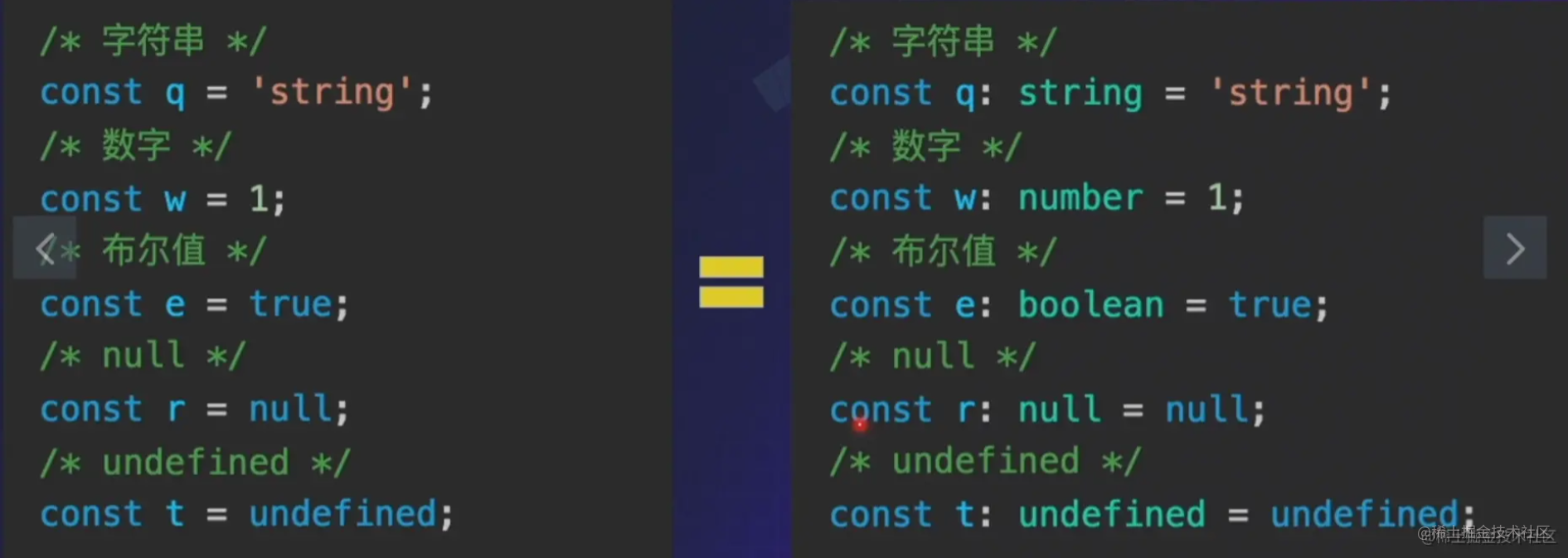# TypeScript学习

TS是JS的一个超集
1、TypeScript有"类"
2、TypeScript有"模块"
3、js没有重载概念，ts有可以重载
4、ts对比js基础类型上，增加了 void/元组等等

Visual Studio Code

1.更稳定
2.可以运行JS 是JS的超集 共存
3.渐进引入function sum(x: number, y: number): number {
return x + y;
}

let mySum: (x: number, y: number) => number = function (x: number, y: number): number {
return x + y;
};

function buildName(firstName: string, lastName?: string) {
if (lastName) {
return firstName + ' ' + lastName;
} else {
return firstName;
}
}
let tomcat = buildName('Tom', 'Cat');
let tom = buildName('Tom');

function push(array: any[], ...items: any[]) {
items.forEach(function(item) {
array.push(item);
});
}
let a = [];
push(a, 1, 2, 3);

function add(x, y) {
return x + y;
}
add("1", "2"); //"12"
科普